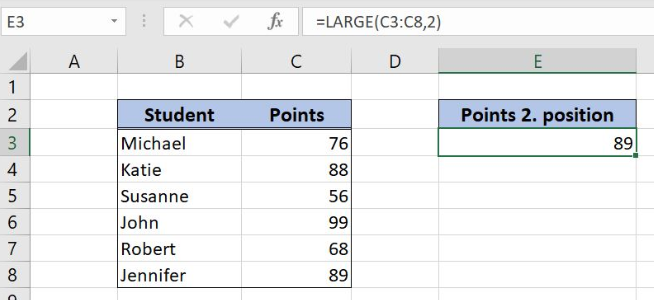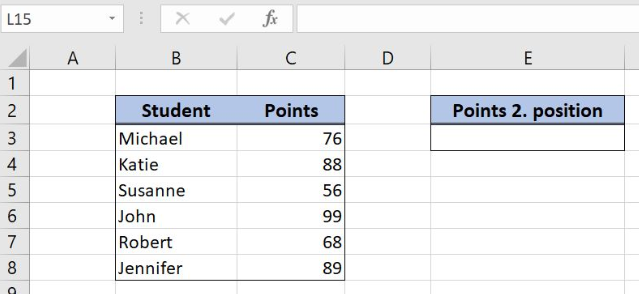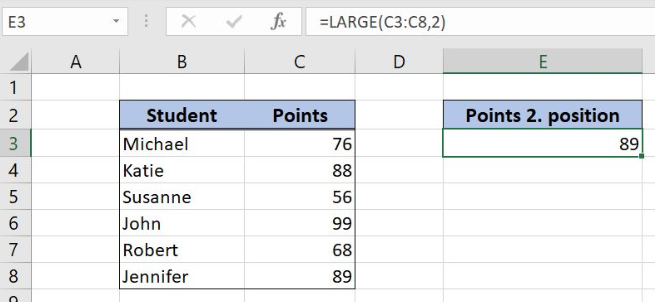Get instant live expert help with Excel or Google Sheets“My Excelchat expert helped me in less than 20 minutes, saving me what would have been 5 hours of work!”

#### Post your problem and you’ll get Expert help in seconds.

Your message must be at least 40 characters
Our professional Expert are available now. Your privacy is guaranteed.

# Excel LARGE Function

Excel allows a user to get a value from a range in a certain position, using the LARGE function. This step by step tutorial will assist all levels of Excel users in getting the Nth value from a range of cells.Figure 1. The result of the LARGE function

## Syntax of the LARGE Formula

The generic formula for the LARGE function is:

`=IF(array, k)`

The parameters of the LARGE function are:

• array – an array of cells where we want to get the Nth value
• k – a position from which we want to get value.

Note that the function ranks an array descendingly, from the greatest value.

## Setting up Our Data for the LARGE Function

Our table consists of 2 columns: “Student” (column B) and “Points” (column C). In the cell E3, we want to get the number of points in the second position.Figure 2. The data for the LARGE function example

## Get a Value in Nth Position Using the LARGE Function

In our example, to get the 2nd value from the Points array.

The formula looks like:

`=LARGE(C3:C8, 2)`

The parameter array is the range C3:C8. The parameter k is 2, as we want to get the value in thesecond position in the array.

To apply the LARGE function, we need to follow these steps:

• Select cell E3 and click on it
• Insert the formula: `=LARGE(C3:C8, 2)`
• Press enter.Figure 3. Using the LARGE function to get the 2nd value in the range

As you can see in Figure 3, the second-ranked point value is 89, so this is the result of the function in the cell E3.

Most of the time, the problem you will need to solve will be more complex than a simple application of a formula or function. If you want to save hours of research and frustration, try our live Excelchat service! Our Excel Experts are available 24/7 to answer any Excel question you may have. We guarantee a connection within 30 seconds and a customized solution within 20 minutes.

### Did this post not answer your question? Get a solution from connecting with the expert.Another blog reader asked this question today on Excelchat:## Subscribe to Excelchat.coAnother blog reader asked this question today on Excelchat: